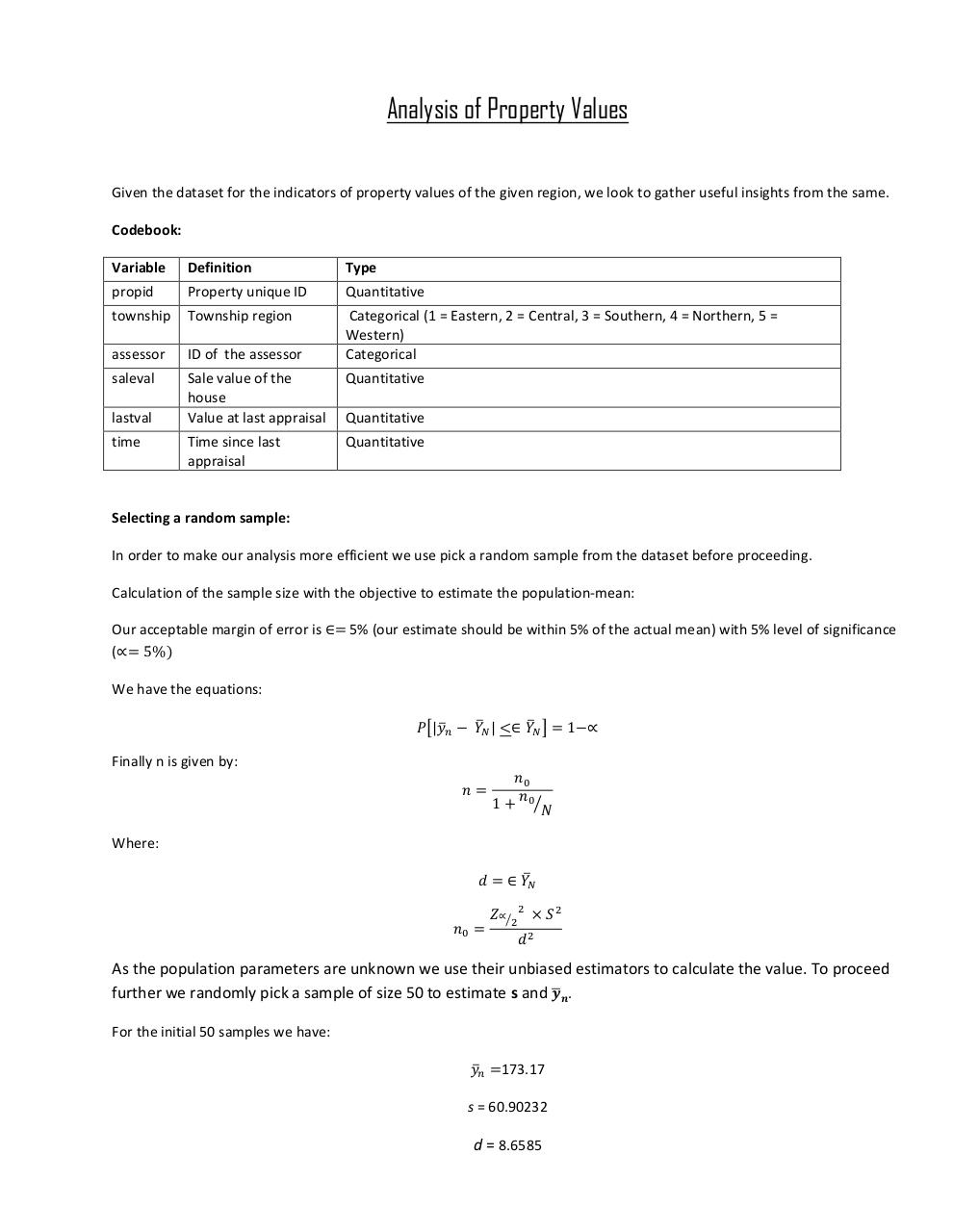# Report.pdfPage 12322

#### Text preview

Analysis of Property Values
Given the dataset for the indicators of property values of the given region, we look to gather useful insights from the same.
Codebook:
Variable

Definition

Type

propid

Property unique ID

Quantitative

township

Township region

assessor

ID of the assessor

Categorical (1 = Eastern, 2 = Central, 3 = Southern, 4 = Northern, 5 =
Western)
Categorical

saleval

Sale value of the
house
Value at last appraisal

Quantitative

Time since last
appraisal

Quantitative

lastval
time

Quantitative

Selecting a random sample:
In order to make our analysis more efficient we use pick a random sample from the dataset before proceeding.
Calculation of the sample size with the objective to estimate the population-mean:
Our acceptable margin of error is ∈= 5% (our estimate should be within 5% of the actual mean) with 5% level of significance
(∝= 5%)
We have the equations:
𝑃[|𝑦̅𝑛 − 𝑌̅𝑁 | &lt;∈ 𝑌̅𝑁 ] = 1−∝
Finally n is given by:
𝑛=

𝑛0
𝑛
1 + 0⁄𝑁

Where:
𝑑 = ∈ 𝑌̅𝑁
𝑛0 =

𝑍∝⁄2 2 × 𝑆 2
𝑑2

As the population parameters are unknown we use their unbiased estimators to calculate the value. To proceed
̅𝒏 .
further we randomly pick a sample of size 50 to estimate s and 𝒚
For the initial 50 samples we have:
𝑦̅𝑛 =173.17
s = 60.90232

d = 8.6585